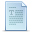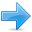# Basic Input/Output

Until now, the example programs of previous sections provided very little interaction with the user, if any at all. Using the standard input and output library, we will be able to interact with the user by printing messages on the screen and getting the user's input from the keyboard.

C++ uses a convenient abstraction called streams to perform input and output operations in sequential media such as the screen or the keyboard. A stream is an object where a program can either insert or extract characters to/from it. We do not really need to care about many specifications about the physical media associated with the stream - we only need to know it will accept or provide characters sequentially.

The standard C++ library includes the header file iostream, where the standard input and output stream objects are declared.

### Standard Output (cout)

By default, the standard output of a program is the screen, and the C++ stream object defined to access it is cout.

cout is used in conjunction with the insertion operator, which is written as << (two "less than" signs).

 ``123`` ``````cout << "Output sentence"; // prints Output sentence on screen cout << 120; // prints number 120 on screen cout << x; // prints the content of x on screen ``````

The << operator inserts the data that follows it into the stream preceding it. In the examples above it inserted the constant string Output sentence, the numerical constant 120 and variable x into the standard output stream cout. Notice that the sentence in the first instruction is enclosed between double quotes (") because it is a constant string of characters. Whenever we want to use constant strings of characters we must enclose them between double quotes (") so that they can be clearly distinguished from variable names. For example, these two sentences have very different results:

 ``12`` ``````cout << "Hello"; // prints Hello cout << Hello; // prints the content of Hello variable ``````

The insertion operator (<<) may be used more than once in a single statement:

 ``1`` ``cout << "Hello, " << "I am " << "a C++ statement";``

This last statement would print the message Hello, I am a C++ statement on the screen. The utility of repeating the insertion operator (<<) is demonstrated when we want to print out a combination of variables and constants or more than one variable:

 ``1`` ``cout << "Hello, I am " << age << " years old and my zipcode is " << zipcode;``

If we assume the age variable to contain the value 24 and the zipcode variable to contain 90064 the output of the previous statement would be:

 ``1`` ``Hello, I am 24 years old and my zipcode is 90064``

It is important to notice that cout does not add a line break after its output unless we explicitly indicate it, therefore, the following statements:

 ``12`` ``````cout << "This is a sentence."; cout << "This is another sentence.";``````

will be shown on the screen one following the other without any line break between them:

This is a sentence.This is another sentence.

even though we had written them in two different insertions into cout. In order to perform a line break on the output we must explicitly insert a new-line character into cout. In C++ a new-line character can be specified as \n (backslash, n):

 ``12`` ``````cout << "First sentence.\n"; cout << "Second sentence.\nThird sentence.";``````

This produces the following output:

First sentence.
Second sentence.
Third sentence.

Additionally, to add a new-line, you may also use the endl manipulator. For example:

 ``12`` ``````cout << "First sentence." << endl; cout << "Second sentence." << endl;``````

would print out:

First sentence.
Second sentence.

The endl manipulator produces a newline character, exactly as the insertion of '\n' does, but it also has an additional behavior when it is used with buffered streams: the buffer is flushed. Anyway, cout will be an unbuffered stream in most cases, so you can generally use both the \n escape character and the endl manipulator in order to specify a new line without any difference in its behavior.

### Standard Input (cin).

The standard input device is usually the keyboard. Handling the standard input in C++ is done by applying the overloaded operator of extraction (>>) on the cin stream. The operator must be followed by the variable that will store the data that is going to be extracted from the stream. For example:

 ``12`` ``````int age; cin >> age;``````

The first statement declares a variable of type int called age, and the second one waits for an input from cin (the keyboard) in order to store it in this integer variable.

cin can only process the input from the keyboard once the RETURN key has been pressed. Therefore, even if you request a single character, the extraction from cin will not process the input until the user presses RETURN after the character has been introduced.

You must always consider the type of the variable that you are using as a container with cin extractions. If you request an integer you will get an integer, if you request a character you will get a character and if you request a string of characters you will get a string of characters.

 ``````// i/o example #include using namespace std; int main () { int i; cout << "Please enter an integer value: "; cin >> i; cout << "The value you entered is " << i; cout << " and its double is " << i*2 << ".\n"; return 0; }`````` ```Please enter an integer value: 702 The value you entered is 702 and its double is 1404.```

The user of a program may be one of the factors that generate errors even in the simplest programs that use cin (like the one we have just seen). Since if you request an integer value and the user introduces a name (which generally is a string of characters), the result may cause your program to misoperate since it is not what we were expecting from the user. So when you use the data input provided by cin extractions you will have to trust that the user of your program will be cooperative and that he/she will not introduce his/her name or something similar when an integer value is requested. A little ahead, when we see the stringstream class we will see a possible solution for the errors that can be caused by this type of user input.

You can also use cin to request more than one datum input from the user:

 ``1`` ``cin >> a >> b;``

is equivalent to:

 ``12`` ``````cin >> a; cin >> b;``````

In both cases the user must give two data, one for variable a and another one for variable b that may be separated by any valid blank separator: a space, a tab character or a newline.

### cin and strings

We can use cin to get strings with the extraction operator (>>) as we do with fundamental data type variables:

 ``1`` ``cin >> mystring;``

However, as it has been said, cin extraction stops reading as soon as if finds any blank space character, so in this case we will be able to get just one word for each extraction. This behavior may or may not be what we want; for example if we want to get a sentence from the user, this extraction operation would not be useful.

In order to get entire lines, we can use the function getline, which is the more recommendable way to get user input with cin:

 ``````// cin with strings #include #include using namespace std; int main () { string mystr; cout << "What's your name? "; getline (cin, mystr); cout << "Hello " << mystr << ".\n"; cout << "What is your favorite team? "; getline (cin, mystr); cout << "I like " << mystr << " too!\n"; return 0; }`````` ```What's your name? Juan Soulie Hello Juan Soulie. What is your favorite team? The Isotopes I like The Isotopes too!```

Notice how in both calls to getline we used the same string identifier (mystr). What the program does in the second call is simply to replace the previous content by the new one that is introduced.

### stringstream

The standard header file <sstream> defines a class called stringstream that allows a string-based object to be treated as a stream. This way we can perform extraction or insertion operations from/to strings, which is especially useful to convert strings to numerical values and vice versa. For example, if we want to extract an integer from a string we can write:

 ``123`` ``````string mystr ("1204"); int myint; stringstream(mystr) >> myint;``````

This declares a string object with a value of "1204", and an int object. Then we use stringstream's constructor to construct an object of this type from the string object. Because we can use stringstream objects as if they were streams, we can extract an integer from it as we would have done on cin by applying the extractor operator (>>) on it followed by a variable of type int.

After this piece of code, the variable myint will contain the numerical value 1204.

 ``````// stringstreams #include #include #include using namespace std; int main () { string mystr; float price=0; int quantity=0; cout << "Enter price: "; getline (cin,mystr); stringstream(mystr) >> price; cout << "Enter quantity: "; getline (cin,mystr); stringstream(mystr) >> quantity; cout << "Total price: " << price*quantity << endl; return 0; }`````` ```Enter price: 22.25 Enter quantity: 7 Total price: 155.75```

In this example, we acquire numeric values from the standard input indirectly. Instead of extracting numeric values directly from the standard input, we get lines from the standard input (cin) into a string object (mystr), and then we extract the integer values from this string into a variable of type int (quantity).

Using this method, instead of direct extractions of integer values, we have more control over what happens with the input of numeric values from the user, since we are separating the process of obtaining input from the user (we now simply ask for lines) with the interpretation of that input. Therefore, this method is usually preferred to get numerical values from the user in all programs that are intensive in user input.Previous:OperatorsIndexNext:Simple control structures